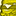QlikView App Dev

Discussion Board for collaboration related to QlikView App Development.

Announcements
QlikWorld 2022, LIVE in Denver CO., May 16-19, 2022. REGISTER NOW TO RECEIVE EARLY BIRD PRICING
cancel
Showing results for
Did you mean:Creator

Reusing variables created in Load Script

Hi,

I am creating variables in my script.  I wanted to know can you utilise those created variables in the same script.

e.g I want to be able to divide two variables I've created

Let Var1 = 'sum(Int1+int2)';

Let Var2 = 'sum(Int3+Int4)';

Can I now divide Var1/var2? in my script and if I can, can you help me with the syntax.

Regards,

1 Solution

Accepted SolutionsPartner

You can utilize the variables in the script, but not those ones. Sum() is not legal in the load script except in a load. If you need to divide the two values, you will need to create them in a load statement, then peek the values to variables, and then divide the variables.

T1:

LOAD Sum(Int1 + Int2) as Sum1,

.....

Group By ....

T2:

Load Sum(Int3 + Int4) as Sum2,

...

Group By ....

Let Var1 = Peek('Sum1', 1, 'T1');

Let Var2 = Peek('Sum2', 1, 'T2');

Let Var3 = Var1 / Var2;

//Optional

DROP Tables T1, T2;

Logic will get you from a to b. Imagination will take you everywhere. - A Einstein
3 RepliesCreator
Author

To add, I now want to create a third variable like:

Let Var3: Var1/Var2;

Or I want to be able to do it in an expression but currently I am not getting the right resultPartner

You can utilize the variables in the script, but not those ones. Sum() is not legal in the load script except in a load. If you need to divide the two values, you will need to create them in a load statement, then peek the values to variables, and then divide the variables.

T1:

LOAD Sum(Int1 + Int2) as Sum1,

.....

Group By ....

T2:

Load Sum(Int3 + Int4) as Sum2,

...

Group By ....

Let Var1 = Peek('Sum1', 1, 'T1');

Let Var2 = Peek('Sum2', 1, 'T2');

Let Var3 = Var1 / Var2;

//Optional

DROP Tables T1, T2;

Logic will get you from a to b. Imagination will take you everywhere. - A EinsteinCreator
Author

Hi Jonathan,

Thanks, that answers my root cause.  I am currently changing my dashboard to one that has a 3 tier architecture and have to bring all my variables I created using the variable overview to the script.

What I have done is just put the Var I created in the script in ' ' because I have various set analysis/sums etc and this seems to work, but gives me incorrect values when I divide variables created this way.

In future, I will use Load Statements for any calculations.Community Browser